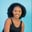Related Tags

pandas
pythons

# How to use the Series.gt() function in pandasMaria Elijah

## Pandas Series.gt() function

The pandas Series.gt() function compares two series element by element to see which one is greater, and it returns a boolean value.

### How does it work?

The function checks element-wise if the Series a is greater than Series b and returns True. Otherwise, it returns False.

### Syntax

Let’s view the syntax of the method:

Series.gt(other, level=None, fill_value=None, axis=0)


### Parameters

• other: This represents the other Series to be compared.

• level: This is an optional parameter. The default is None. It specifies the level (in the case of multilevel) to count along.

• fill_value: This represents the value to replace the NaN value. If data in both corresponding Series indexes is NaN, then the result of filling at that specific index will be missing.

• axis: This specifies on which axis the operation is to be performed. 0 indicates rows and 1 indicates column.

### Code example

The following code demonstrates how to use the Series.gt() function in pandas:

import pandas as pd
import numpy as np

# create Series
a = pd.Series([7, 12, 9, np.nan, 23, 40, np.nan])

b = pd.Series([10, np.nan, 28, 45, 73, 7, np.nan])

# value to fill nan
replace_nan = 3

# finds the greater element in each Series using Series.gt()
print(a.gt(b, fill_value=replace_nan))
Try it out!

### Code explanation

In the code above:

• Lines 1 and 2: We import the libraries pandas and numpy.

• Lines 5: We create a Series a.

• Lines 7: We create a series b.

• Lines 10: We create a variable replace_nan and assign a value to replace the NaN values in the Series.

• Line 13: We compare the elements in Series a and b using the gt() function.

Note: If the element in both corresponding Series indexes is NaN, then the replace_nan value won’t be used.

RELATED TAGS

pandas
pythons

CONTRIBUTORMaria Elijah
RELATED COURSES

View all Courses

Keep Exploring

Learn in-demand tech skills in half the time